李宏毅+机器学习_李宏毅机器学习深度学习视频 - CSDN
• 关于“一个属性有几个固定选项，怎么表达”的问题，数据采用one-hot，每个选项独立成一个属性，loss用交叉熵计算，这样更科学。这里不用过多操心，数据给出已经处理好了。 有一段可能是助教给的参考代码，不过...
关于“一个属性有几个固定选项，怎么表达”的问题，数据采用one-hot，每个选项独立成一个属性，loss用交叉熵计算，这样更科学。这里不用过多操心，数据给出已经处理好了。

有一段可能是助教给的参考代码，不过参考代码有些漏洞（在代码下方贴出），需要修改下，然后还有一定的调参提升空间。

参考代码如下：

import os, sys
import numpy as np
from random import shuffle
import argparse
from math import log, floor
import pandas as pd

# If you wish to get the same shuffle result
# np.random.seed(2401)

X_train = np.array(X_train.values)
Y_train = np.array(Y_train.values)
X_test = np.array(X_test.values)

return (X_train, Y_train, X_test)

def _shuffle(X, Y):
randomize = np.arange(len(X))
np.random.shuffle(randomize)
return (X[randomize], Y[randomize])

def normalize(X_all, X_test):
# Feature normalization with train and test X
X_train_test = np.concatenate((X_all, X_test))
mu = (sum(X_train_test) / X_train_test.shape)
sigma = np.std(X_train_test, axis=0)
mu = np.tile(mu, (X_train_test.shape, 1))
sigma = np.tile(sigma, (X_train_test.shape, 1))
X_train_test_normed = (X_train_test - mu) / sigma

# Split to train, test again
X_all = X_train_test_normed[0:X_all.shape]
X_test = X_train_test_normed[X_all.shape:]
return X_all, X_test

def split_valid_set(X_all, Y_all, percentage):
all_data_size = len(X_all)
valid_data_size = int(floor(all_data_size * percentage))#这个比例反了。

X_all, Y_all = _shuffle(X_all, Y_all)

X_train, Y_train = X_all[0:valid_data_size], Y_all[0:valid_data_size]
X_valid, Y_valid = X_all[valid_data_size:], Y_all[valid_data_size:]

return X_train, Y_train, X_valid, Y_valid

def sigmoid(z):
res = 1 / (1.0 + np.exp(-z))
return np.clip(res, 1e-8, 1-(1e-8))

def valid(w, b, X_valid, Y_valid):
valid_data_size = len(X_valid)

z = (np.dot(X_valid, np.transpose(w)) + b)
y = sigmoid(z)
y_ = np.around(y)
result = (np.squeeze(Y_valid) == y_)
print('Validation acc = %f' % (float(result.sum()) / valid_data_size))
return

def train(X_all, Y_all, save_dir):

# Split a 10%-validation set from the training set
valid_set_percentage = 0.1
X_train, Y_train, X_valid, Y_valid = split_valid_set(X_all, Y_all, valid_set_percentage)

# Initiallize parameter, hyperparameter
w = np.zeros((106,))
b = np.zeros((1,))
l_rate = 0.1
batch_size = 32
train_data_size = len(X_train)
step_num = int(floor(train_data_size / batch_size))
epoch_num = 1000
save_param_iter = 50

# Start training
total_loss = 0.0
for epoch in range(1, epoch_num):
# Do validation and parameter saving
if (epoch) % save_param_iter == 0:
print('=====Saving Param at epoch %d=====' % epoch)
if not os.path.exists(save_dir):
os.mkdir(save_dir)
np.savetxt(os.path.join(save_dir, 'w'), w)
np.savetxt(os.path.join(save_dir, 'b'), [b,])
print('epoch avg loss = %f' % (total_loss / (float(save_param_iter) * train_data_size)))
total_loss = 0.0
valid(w, b, X_valid, Y_valid)

# Random shuffle
X_train, Y_train = _shuffle(X_train, Y_train)

# Train with batch
for idx in range(step_num):
X = X_train[idx*batch_size:(idx+1)*batch_size]
Y = Y_train[idx*batch_size:(idx+1)*batch_size]

z = np.dot(X, np.transpose(w)) + b
y = sigmoid(z)

cross_entropy = -1 * (np.dot(np.squeeze(Y), np.log(y)) + np.dot((1 - np.squeeze(Y)), np.log(1 - y)))
total_loss += cross_entropy

w_grad = np.sum(-1 * X * (np.squeeze(Y) - y).reshape((batch_size,1)), axis=0)
b_grad = np.sum(-1 * (np.squeeze(Y) - y))

# SGD updating parameters
w = w - l_rate * w_grad
b = b - l_rate * b_grad

return

def infer(X_test, save_dir, output_dir):
test_data_size = len(X_test)

# predict
z = (np.dot(X_test, np.transpose(w)) + b)
y = sigmoid(z)
y_ = np.around(y)

print('=====Write output to %s =====' % output_dir)
if not os.path.exists(output_dir):
os.mkdir(output_dir)
output_path = os.path.join(output_dir, 'log_prediction.csv')
with open(output_path, 'w') as f:
f.write('id,label\n')
for i, v in  enumerate(y_):
f.write('%d,%d\n' %(i+1, v))

return

def main(opts):
X_all, Y_all, X_test = load_data(opts.train_data_path, opts.train_label_path, opts.test_data_path)
# Normalization
X_all, X_test = normalize(X_all, X_test)

# To train or to infer
if opts.train:
train(X_all, Y_all, opts.save_dir)
elif opts.infer:
infer(X_test, opts.save_dir, opts.output_dir)
else:
return

if __name__ == '__main__':
parser = argparse.ArgumentParser(description='Logistic Regression with Gradient Descent Method')
dest='train', help='Input --train to Train')
dest='infer', help='Input --infer to Infer')
default='feature/X_train', dest='train_data_path',
help='Path to training data')
default='feature/Y_train', dest='train_label_path',
help='Path to training data\'s label')
default='feature/X_test', dest='test_data_path',
help='Path to testing data')
default='logistic_params/', dest='save_dir',
help='Path to save the model parameters')
default='logistic_output/', dest='output_dir',
help='Path to save the model parameters')
opts = parser.parse_args()
main(opts)

代码错误有几点：

1.每一个epoch中，用于计算cross_entropy从而统计进total_loss的数据量，是step_num*batch_size，小于train_data_size。而ta

它用的是train_data_size。

这两个数据对比：结果证实，确实有点偏差，不过因为他只是打印，不是训练过程，所以看起来也没影响结果。

2.split_valid_set中，训练集和验证集划分错了，用验证集的比例，提取了训练集。

这个也很明显，会对结果产生显著影响

3.感谢网友weixin_42183288提醒，不过此处确实没错：交叉熵隐含了sum操作！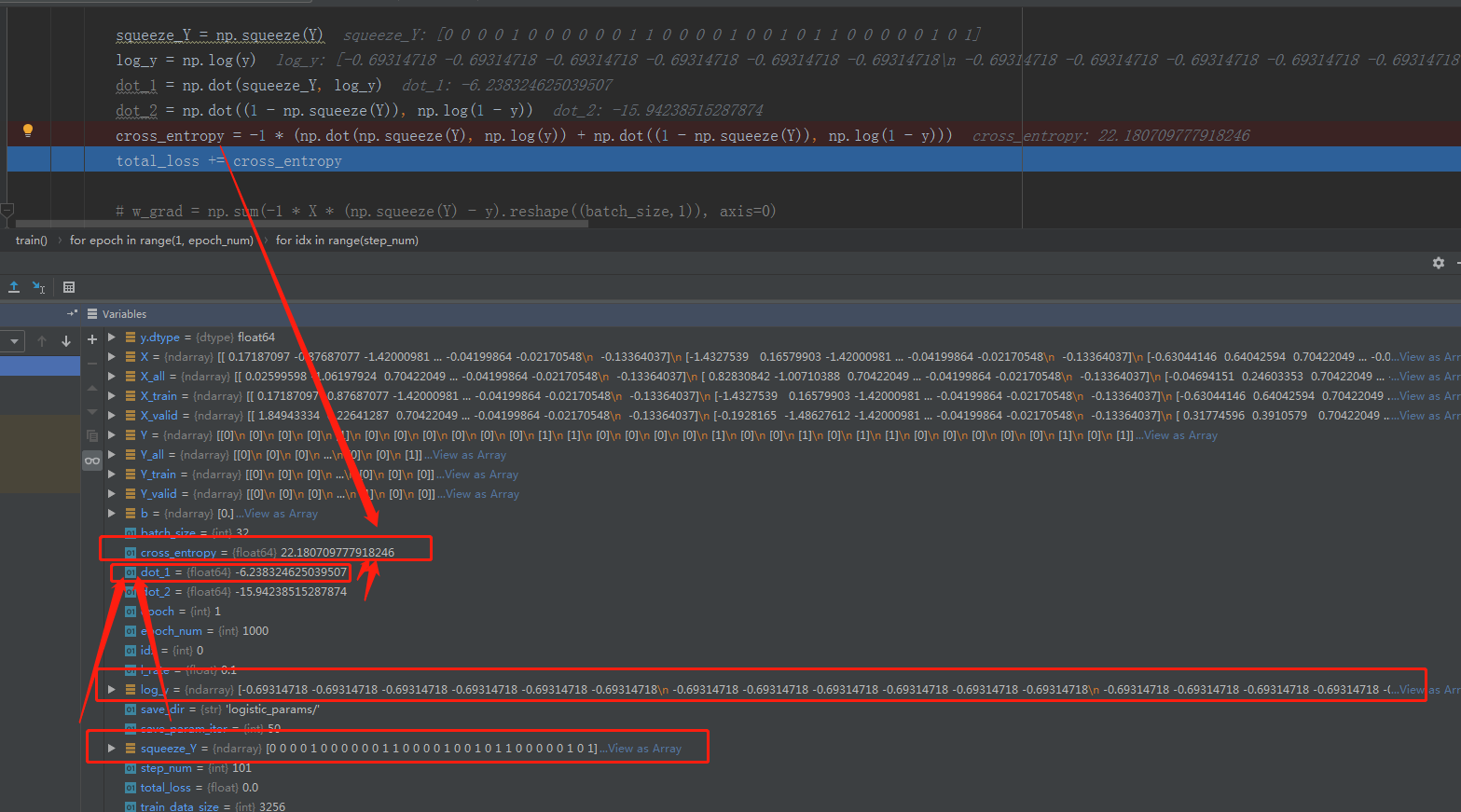print('epoch avg loss = %f' % (total_loss / (float(save_param_iter) * train_data_size)))

从这句打印也看得出，loss确实是所有样本的和，然后才除以迭代epoch次数和data_size，平均到每个样本。

但是打印归打印，这句不是也说了是avg loss嘛！实际的total loss，还是针对batch的sum。

其实这根本不是应该纠结的点！！！这可能是接触代码不多的新手都会碰到的疑惑：

“课程中是除以m，也就是mean”

“loss要用sum还是mean？”

“为什么我用mean比用sum感觉要更准一点？”

本质上，这是没区别的，梯度下降，下降的是什么？loss的值！具体loss或者w的大小，只与计算机对小数的表达能力有关（这个角度看，mean反而不如sum准），而不太可能上升到模型准确度差异的高度！教科书是为了示意，如果他想精准地表达梯度，当然要除以m，可是实际计算当中，区别不大。

            w_grad = np.mean(-1 * X * (np.squeeze(Y) - y).reshape((batch_size,1)), axis=0)
b_grad = np.mean(-1 * (np.squeeze(Y) - y))

# SGD updating parameters
w = w - l_rate * w_grad
b = b - l_rate * b_grad

4.还有个什么错误我忘了。。。。

上传kaggle，这是两条分数分段线：

0.84952高分

0.84215及格

验证集分配错误的，也够及格

0.84803

优化的分配比例的：

        X_valid,Y_valid = X_all[0:valid_data_size],Y_all[0:valid_data_size]
X_train,Y_train = X_all[valid_data_size:],Y_all[valid_data_size:]


0.85159

下边是调整其他参数，batch_size和epoch等：

batch_size提升到64，epoch到5000

0.85356

batch_size提升到64，epoch到5000，l_rate到0.5

0.84201

batch_size提升到64，epoch到5000，l_rate改到0.2，

0.85036

比0.5要强，不过还是比0.1差些，也许有运气成分，每次训练也不一样。

batch_size提升到64，epoch到5000，l_rate改到0.05，

还有训练，集和验证集的比例可以调：

batch_size提升到64，epoch到5000，l_rate改到0.05，验证集改到0.3（默认0.1）

validation acc = 0.851761

实际分数0.85417目前最优，可能说明之前的有过拟合现象。

其他不动，验证集比例改回0.1重新测

validation acc = 0.860258
分数：0.85417，居然一样

本地validation  acc更高一些，实际分数还一样，是有一点过拟合吧？？！！

调了一下记录，可以看到倒数第二次，虽然公开的也是0.85417，和最后一次一样，但是最后一次在private衰减到了0.84891，证明最后一次确实比倒数第二次过拟合。

但是过拟合和过拟合也不一样，有两种过拟合，这里说明最后一次是针对线上公开成绩的过拟合，private set发生衰减。

前边说的是针对验证集的过拟合，线上答案发生衰减。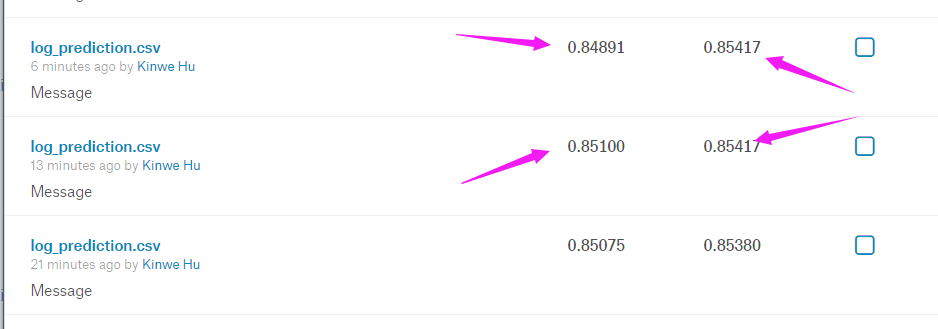紫色字体不算绝对严谨，因为需要更细致的测试对比，每次训练本来就有微小的差距。这里主要讨论可能存在的问题和优化思路，因为时间关系和运算量的关系，就不在这纠结了，先结了。

                w_s_gra = np.zeros(106)
b_s_gra = np.zeros(1)

for idx in range(step_num):
X = X_train[idx*batch_size:(idx+1)*batch_size]
Y = Y_train[idx*batch_size:(idx+1)*batch_size]

z = np.dot(X,np.transpose(w))+b

y = sigmoid(z)

cross_entropy = -1*(np.dot(np.squeeze(Y),np.log(y))+np.dot(1-np.squeeze(Y),np.log(1-y)))
total_loss += cross_entropy



可能有编辑错的地方，凑合看吧，实在懒得多写了，这个csdn现在真难用，拼音打一半就自动换行了。


展开全文机器学习 逻辑回归 代码
• VIDEO 【1】Learning Map（学习导图） blog pdf video 【2】Regression：Case Study ；回归：案例研究 blog pdf video 【3】Gradient Descent ；梯度下降 blog pdf video 【4

今天收到了第一笔赞赏，非常感谢，也很惊喜，o(￣▽￣)ブ

时间
赞赏者
赞赏留言
赞赏数额

2019.7.18
奔跑
感谢你整理Hong-yi Lee老师讲解的机器学习的资料~
5元

2019.7.27
Eivind
谢谢
1元

2019.11.22
*
加油
10元

2019.11.03
比球还圆
谢谢你的工作对我很有帮助
20元

TOPIC
CONTENTS
BLOG
PDF
VIDEO

【1】Learning Map（学习导图）blog
pdf
video

【2】Regression：Case Study ；回归：案例研究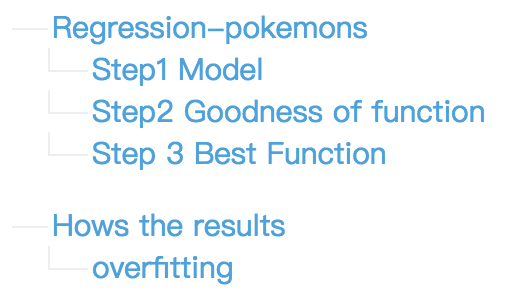blog
pdf
video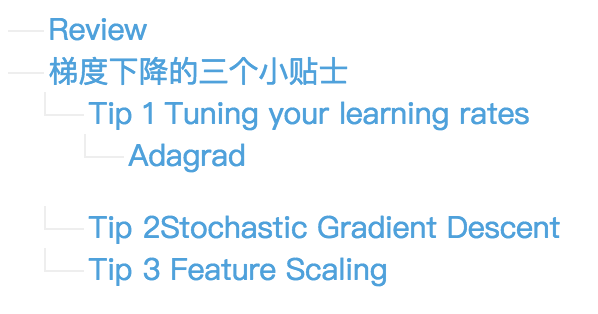blog
pdf
video

【4】Where does the error come from? ；误差分析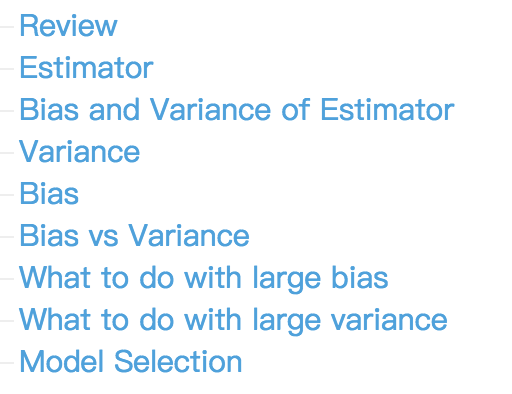blog
pdf
video

【5】Classification: Probabilistic Generative Model；分类：概率生成模型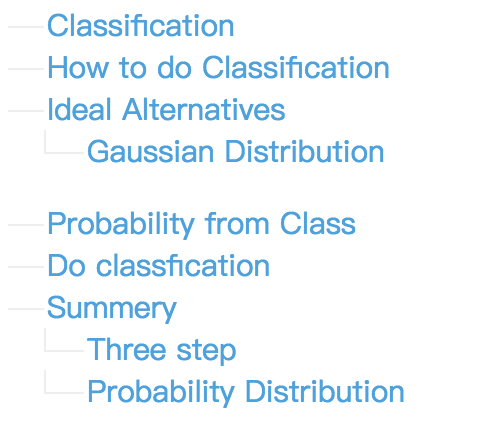blog
pdf
video

【6】Classification: Logistic Regression；Logistic回归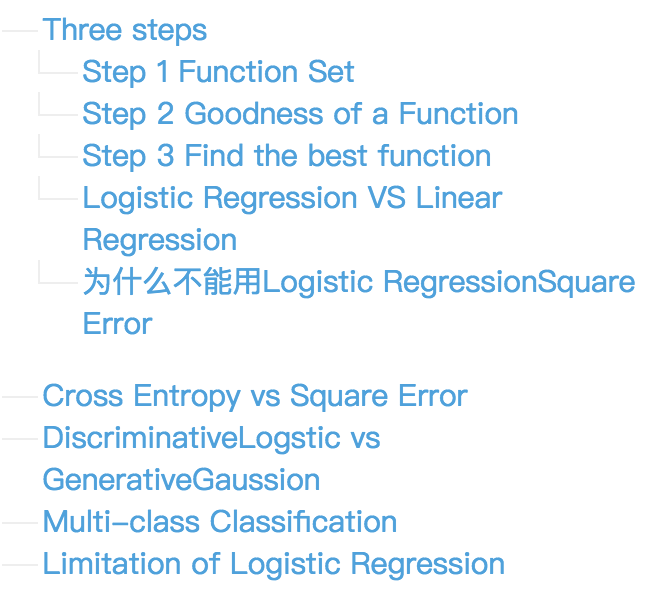blog
pdf
video

【7】Brief Introduction of Deep Learning；深度学习简介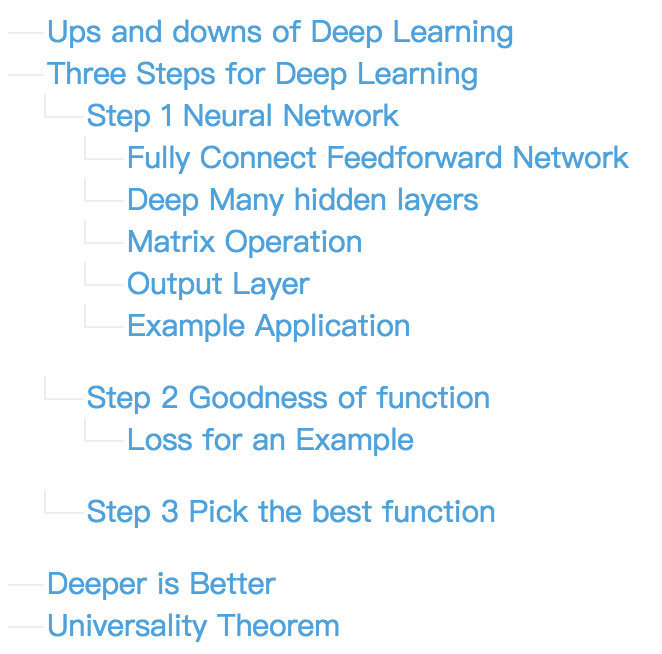blog
pdf
video

【8】Backpropagation；反向传播算法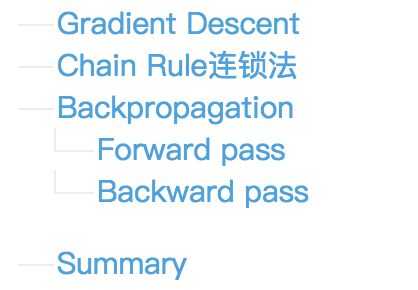blog
pdf
video

【9】“Hello world” of deep learning；初探深度学习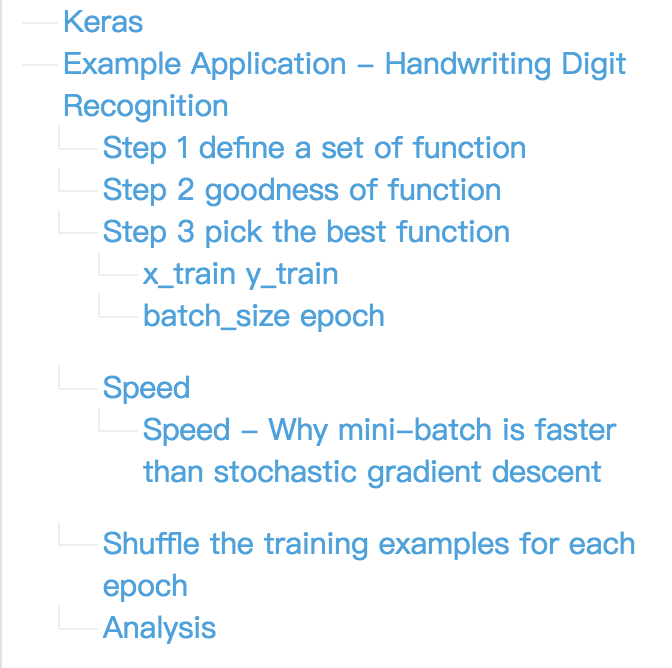blog
pdf
video

【10】Tips for Deep Learning；深度学习小贴士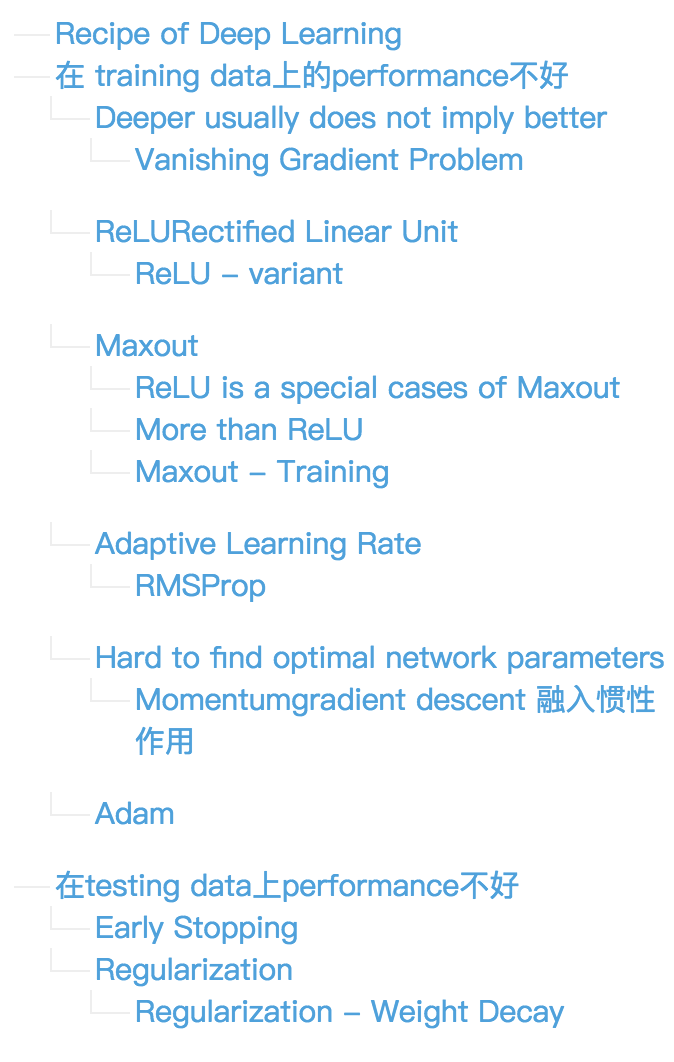blog
pdf
video

【11】Convolutional Neural Network；卷积神经网络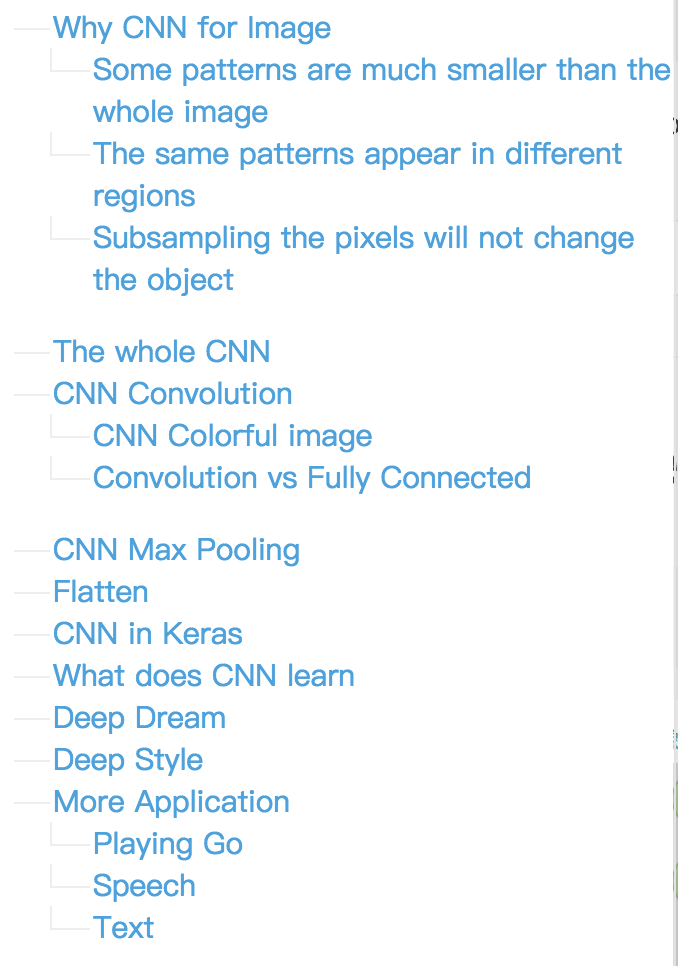blog
pdf
video

【12】Why Deep Learning? ; 为什么是深度学习？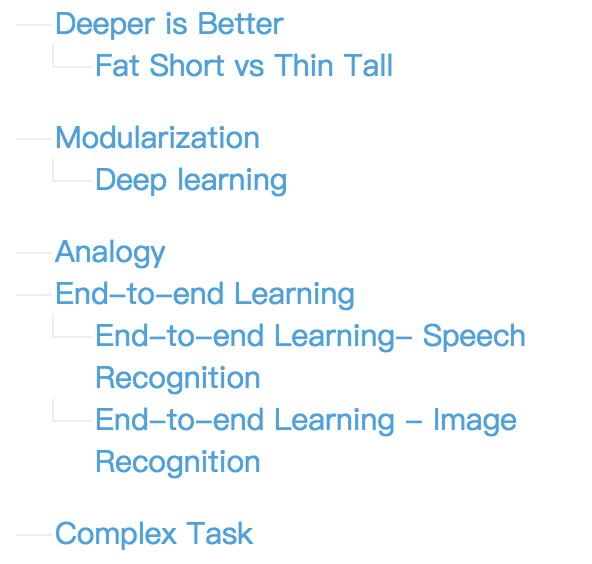blog
pdf
video

【13】Semi-supervised Learning ;半监督学习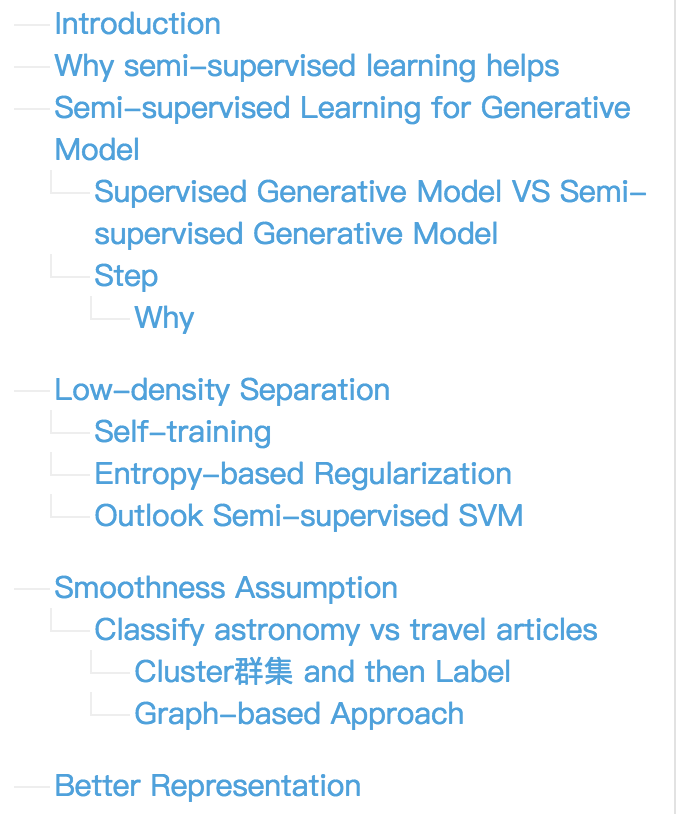blog
pdf
video

【14】Unsupervised Learning: Linear Dimension Reduction；线性降维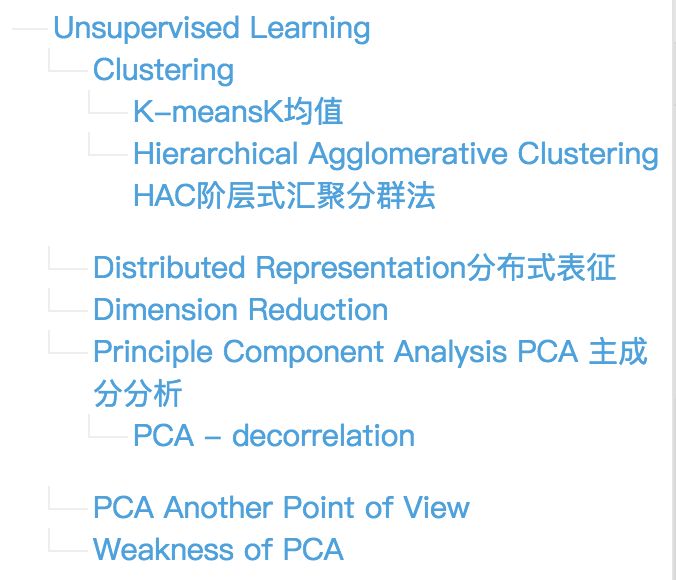blog
pdf
video

【15】Unsupervised Learning: Word Embedding；无监督学习：词嵌入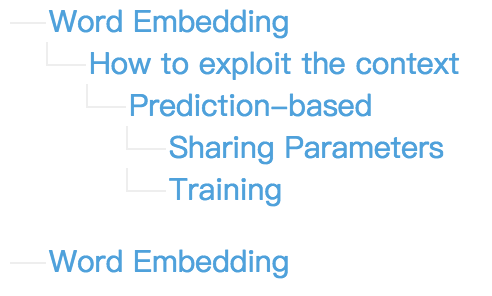blog
pdf
video

【16】Unsupervised Learning: Neighbor Embedding；无监督学习：邻域嵌套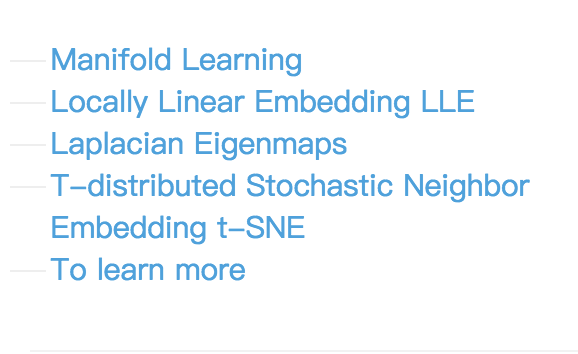blog
pdf
video

【17】Unsupervised Learning: Deep Auto-encoder；无监督学习：深度自动编码器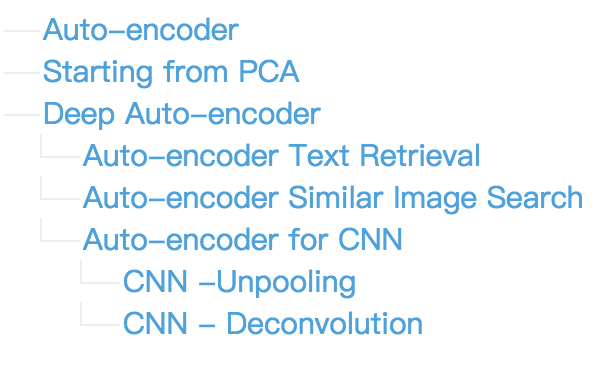blog
pdf
video

【18】Deep Generative Model-part 1：深度生成模型-part 1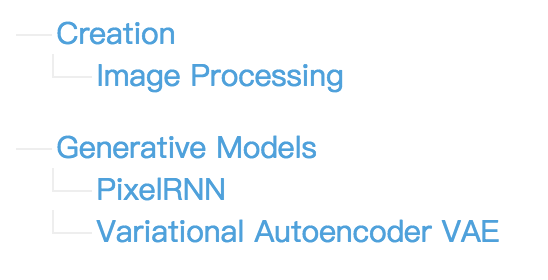blog
pdf
video

【19】Deep Generative Model-part 2：深度生成模型-part 2blog
pdf
video

【20】Deep Generative Model-part 3：深度生成模型-part 3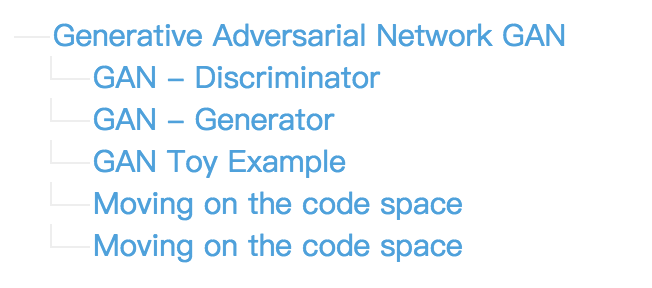blog
pdf
video

【21】Transfer Learning part 1 ; 迁移学习 part 1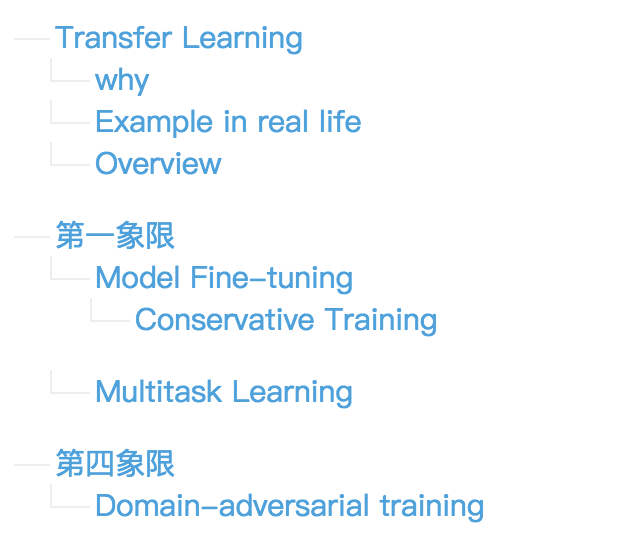blog
pdf
video

【22】Transfer Learning part 2；迁移学习 part 2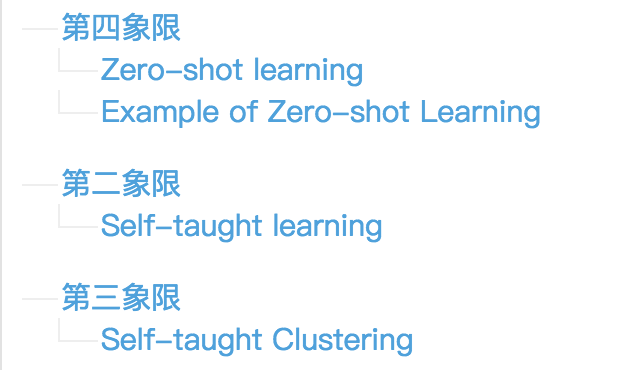blog
pdf
video

【23】Support Vector Machine；支持向量机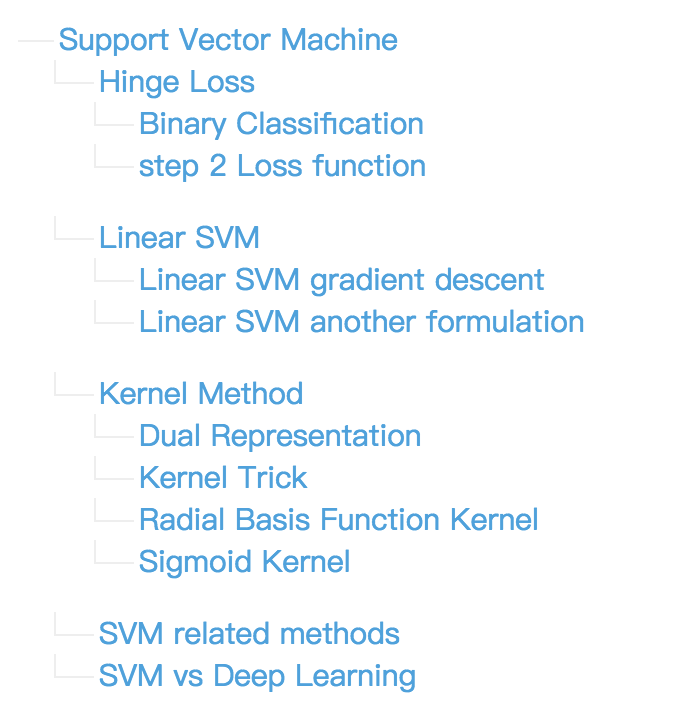blog
pdf
video

【24】introduction of Structured Learning;结构化学习介绍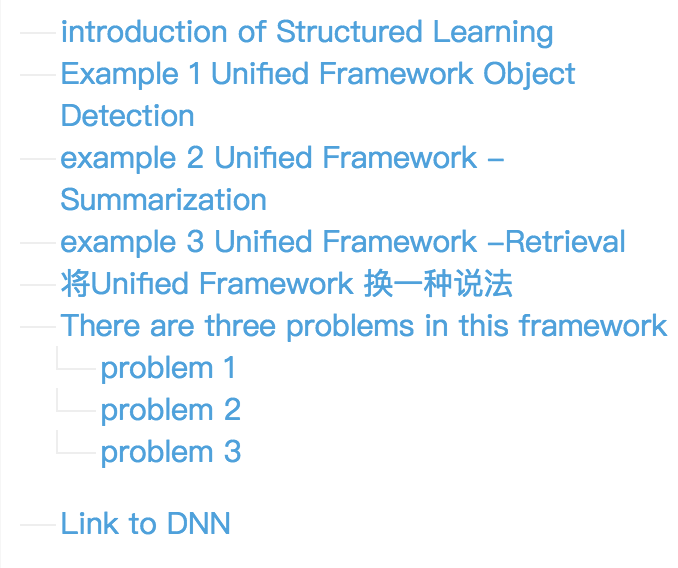blog
pdf
video

【25】Structured Linear Model；结构化预测-线性模型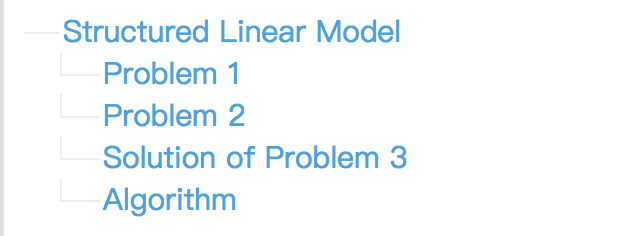blog
pdf
video

【26】Structured Support Vector Machine part 1;结构化支持向量机part 1blog
pdf
video

【27】Structured SVM part 2；结构化支持向量机 part 2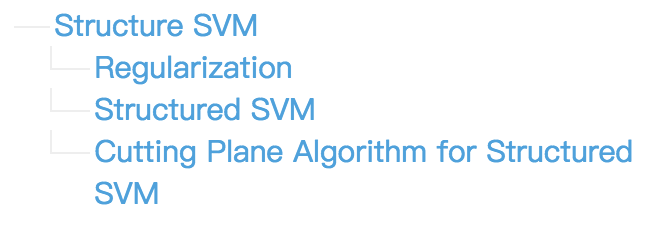blog
pdf
video

【28】Structured SVM part 3 ;结构化支持向量机 part 3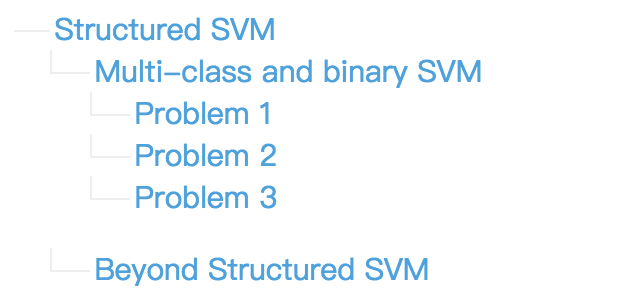blog
pdf
video

【29】Sequence Labeling Problem part 1;结构化预测-序列标记 part 1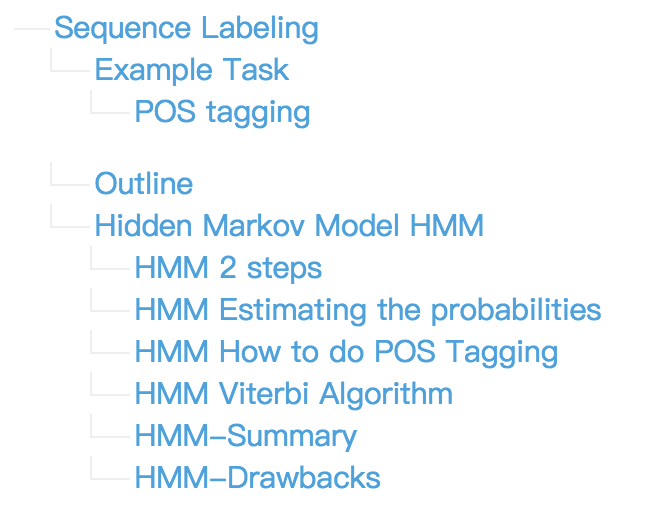blog
pdf
video

【30】Sequence Labeling Problem part 2 ;结构化预测-序列标记 part 2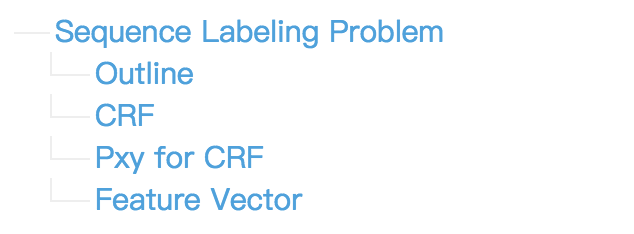blog
pdf
video

【31】Sequence Labeling Problem part 3 ;结构化预测-序列标记 part 3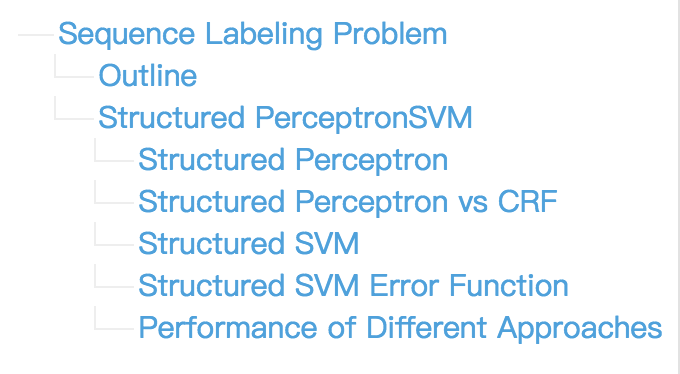blog
pdf
video

【32】Recurrent Neural Network part 1;循环神经网络 part 1blog
pdf
video

【33】Recurrent Neural Network part 2;循环神经网络 part 2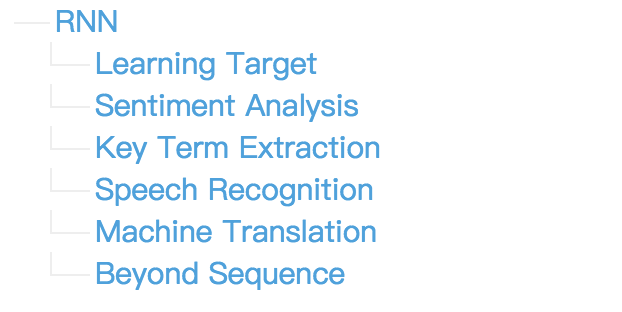blog
pdf
video

【34】Recurrent Neural Network part 3;循环神经网络 part 3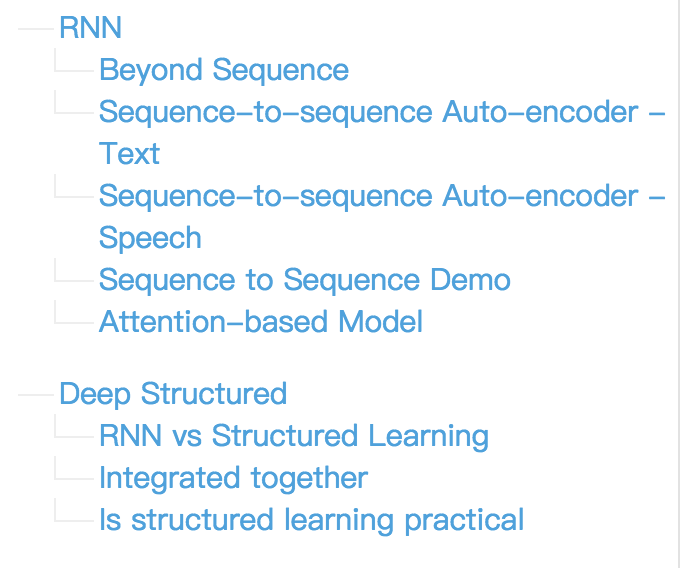blog
pdf
video

【35】Ensemble；集成方法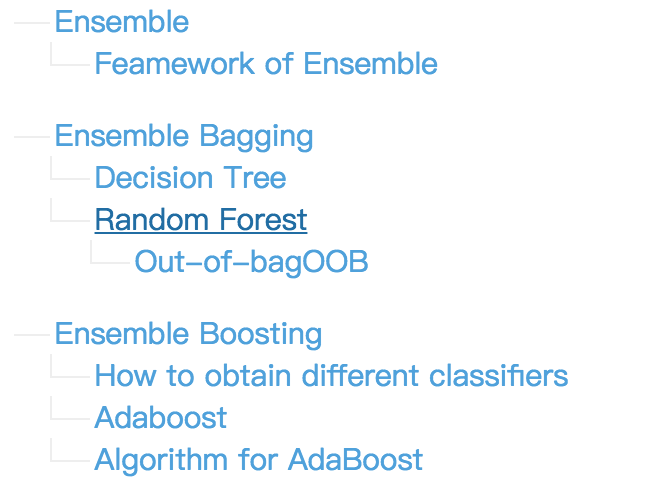blog
pdf
video

【36】Ensemble part 2；集成方法 part 2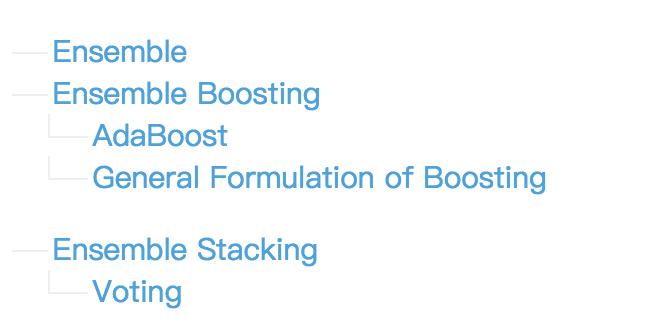blog
pdf
video

【37】Deep Reinforcement Learning;深度增强学习入门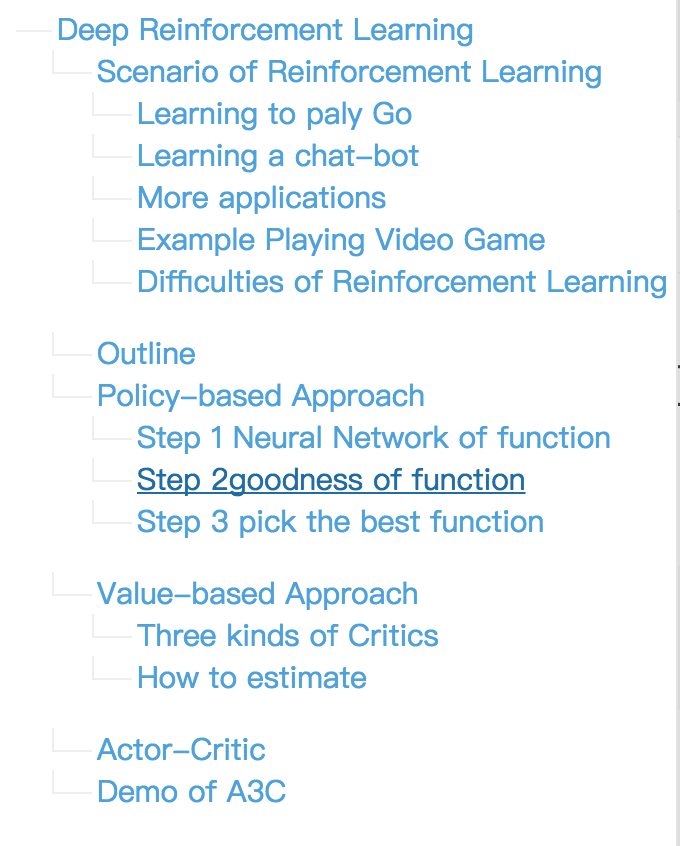blog
pdf
video

【38】李宏毅机器学习课程回顾 + 接下来的学习声明blog

【39】李宏毅机器学习课程从这里开始
本文
blog

相关链接：
课程主页：http://speech.ee.ntu.edu.tw/~tlkagk/courses_ML16.html
PPT打包下载：http://speech.ee.ntu.edu.tw/~tlkagk/courses/ML_2016/all.rar展开全文机器学习 深度学习 笔记
• 机器学习相关岗位在这个人工智能时代还是非常吃香的，经常听到别人几十万、上百万年薪，十分羡慕。在网上搜罗了几份机器学习的教学视频，在这里分享给大家。 更多资源和优质文章，欢迎关注公众号：代码视界。 ...
机器学习相关岗位在这个人工智能时代还是非常吃香的，经常听到别人几十万、上百万年薪，十分羡慕。在网上搜罗了几份机器学习的教学视频，在这里分享给大家。
更多资源和优质文章，欢迎关注公众号：代码视界。

Python3入门机器学习-经典算法与应用
链接: https://pan.baidu.com/s/1NvoRVZbzjGvUzVqmH-RTXQ 提取码: s3fc 复制这段内容后打开百度网盘手机App，操作更方便哦

吴恩达/李宏毅机器学习教学视频

吴恩达和李宏毅两位老师的机器学习教学视频获取方式：
关注公众号：代码视界，回复“机器学习”获取下载地址。

机器学习算法地图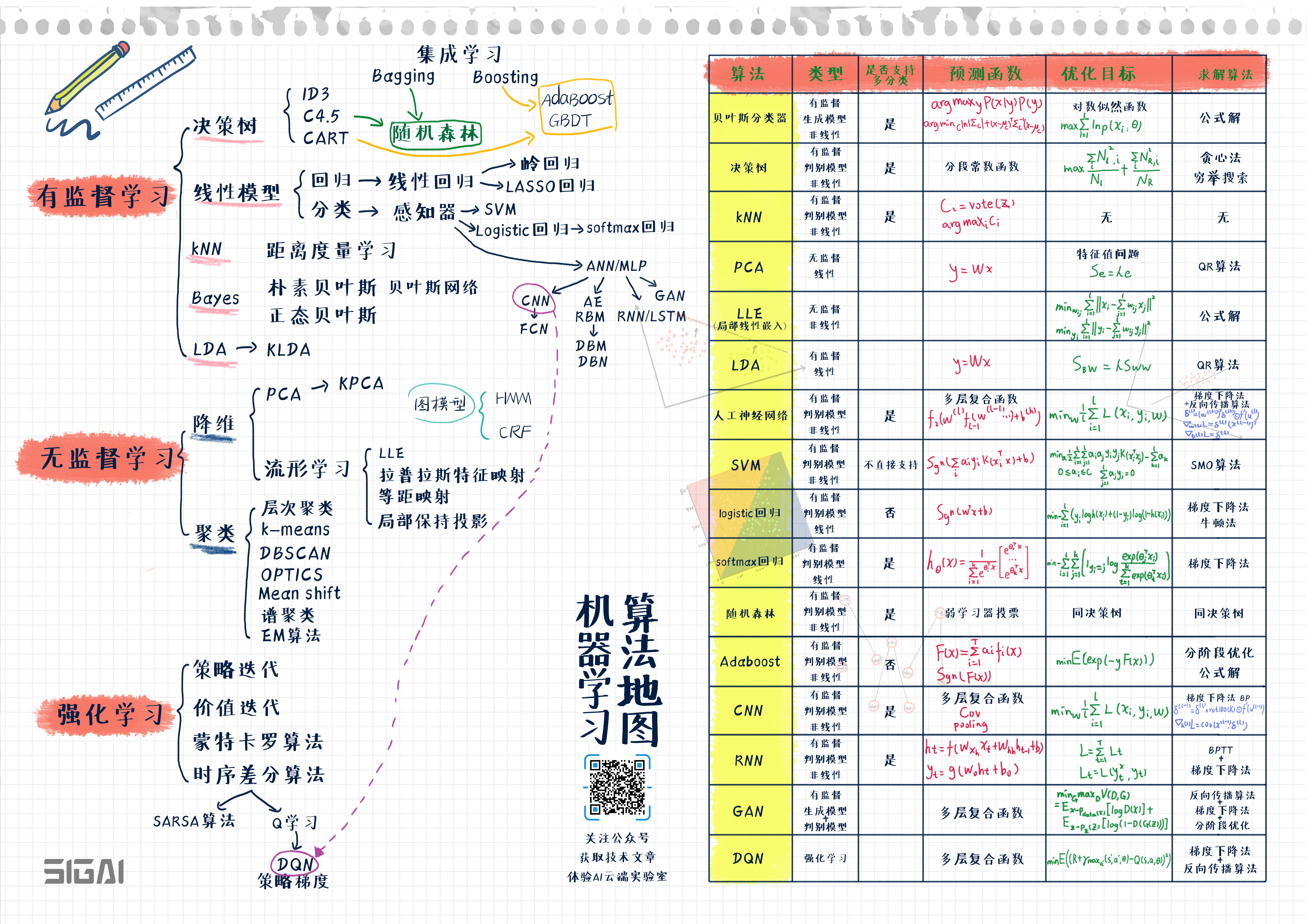展开全文机器学习 吴恩达机器学习 机器学习算法地图
• 李宏毅 机器学习 课程作业代码李宏毅 机器学习 课程作业代码李宏毅 机器学习 课程作业代码李宏毅 机器学习 课程作业代码李宏毅 机器学习 课程作业代码李宏毅 机器学习 课程作业代码李宏毅 机器学习 课程作业代码...
• ## ⭐ 李宏毅2020机器学习资料汇总

万次阅读 多人点赞 2020-08-14 18:52:48
前言 可能受到新冠病毒的影响，台大也开始了...和吴恩达的CS229机器学习相比，中文版本的机器学习显得亲民了许多，李宏毅机器学习是英文的ppt+中文讲解，非常有利于大家入门。吴恩达的CS229中偏向于传统机器学习……
更新进度：■■■■□□□□□□□□□□□□□□□□|25%

文章目录前言储备知识资料学习路线 作业笔记
前言
可能受到新冠病毒的影响，台大也开始了网课教学。李宏毅上传了2020版本的机器学习视频，可以说是非常好的学习资料（尽管其中多数都是2017、2019的视频，但有部分更新）。
和吴恩达的CS229机器学习相比，中文版本的机器学习显得亲民了许多，李宏毅的机器学习是英文的ppt+中文讲解，非常有利于大家入门。吴恩达的CS229中偏向于传统机器学习（线性回归、逻辑回归、Naive Bayes、决策树、支持向量机等），李宏毅2020版本的机器学习中除了最前面的回归、分类，后面更多篇幅涉及卷积神经网络（CNN）、循环神经网络（RNN）、强化学习（RL）等深度学习的内容，不过，似乎b站上也有他的深度学习的课程，具体差异此后再说。
2020年版与2017年版、2019年版较为类似，不同的是2019年版用到了keras这一深度学习框架，而2020年版用到了pytorch。近年来，pytorch在计算机会议论文中占比也逐渐增加。鉴于博主最近做课题需要学习pytorch，李宏毅的2020年版机器学习资料确实非常合适。
李宏毅的机器学习中多处用到了宝可梦（神奇宝贝）、凉宫春日等二次元元素进行举例，对于博主这个伪·二次元来说，学习上没有太大困难。虽然宝可梦、凉宫春日等例子并不影响你的学习，但是博主觉得：如果对它们完全不了解的话，去理解一个陌生的东西可能会比理解猫猫狗狗困难（笑）。对于二次元来说，倒是有种亲切感。
这个教学视频里面还用到一些游戏举例子，可以说是一个非常有趣的机器学习视频，一旦打开可能就会忍不住学下去（实际上大部分人都是收藏，想着以后再看，然后再也没看过） 。有条件的话，建议大家系统学习。博主之前都是需求驱动型学习，导致很多东西都是一知半解。目前看的这个视频带来了一些新的思考，比如梯度下降的原理、验证集和测试集的正确使用方法等，很有帮助。

储备知识
Q：我没有学习过机器学习，需要有什么储备知识？
A：

高等数学：涉及导数、偏微分、泰勒展开等，建议系统学习。
线性代数：涉及向量、协方差矩阵等，建议系统学习。
概率统计：涉及贝叶斯公式、高斯分布、极大似然估计等，夸张点说，机器学习本质是概率统计。建议先学习高数、线性代数，再系统学习。
计算机相关：python，jupyter notebook，anaconda，pyenv，github，kaggle，linux基本指令

资料

李宏毅的课程网页：点击此处跳转
b站有全套视频的搬运：BV1JE411g7XF，也可以点击此处跳转
2020版课后作业范例和作业说明：点击此处跳转
附上别人的github学习笔记：点击此处跳转

学习路线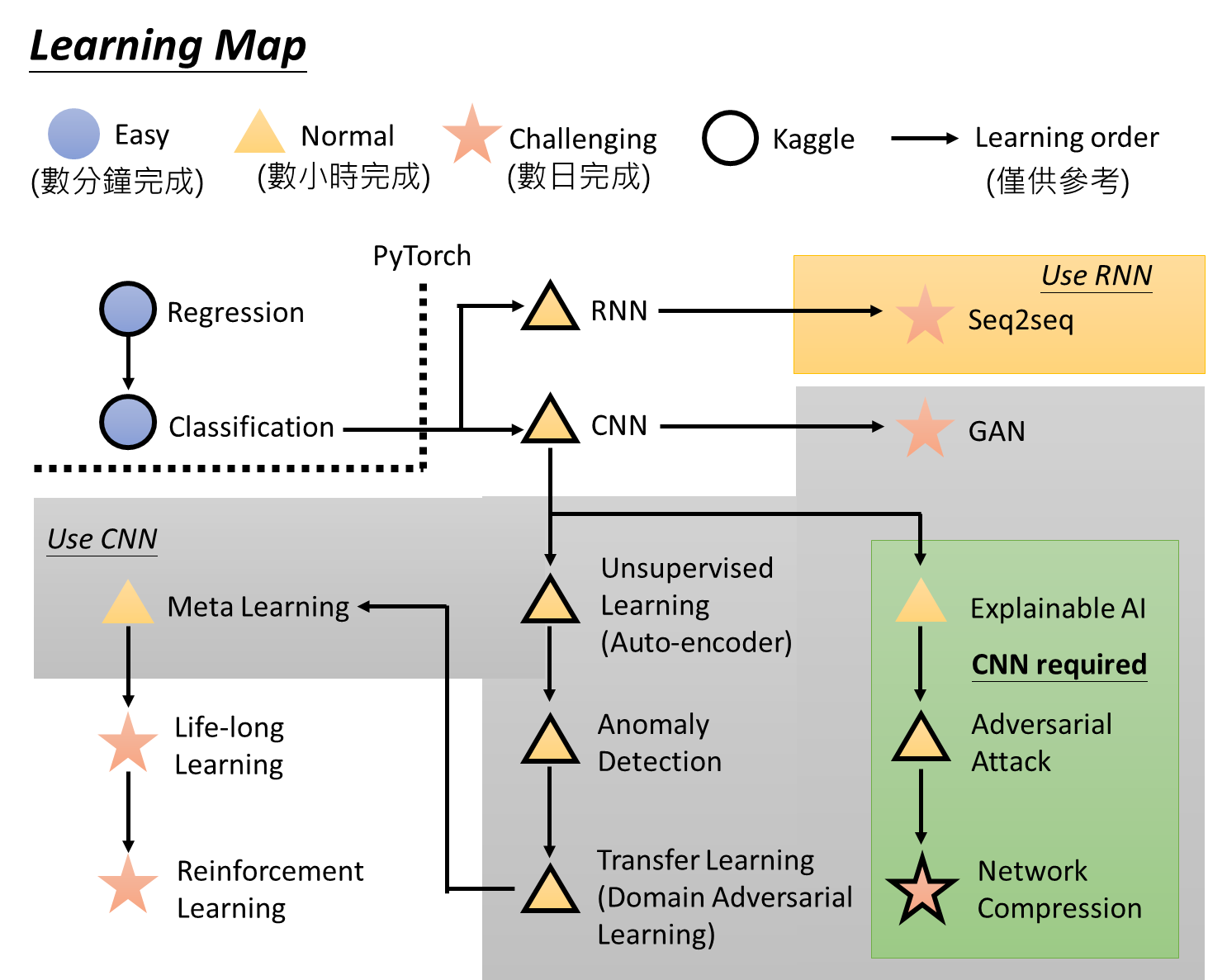这一张可以在李宏毅的课程首页上找到，点开b站视频p1可以了解详情。每一个符号代表一个作业，用到pytorch的框架，博主后续可能会更新自己的作业进度（敦促自己赶紧学习），作业提交主要都在kaggle这个平台。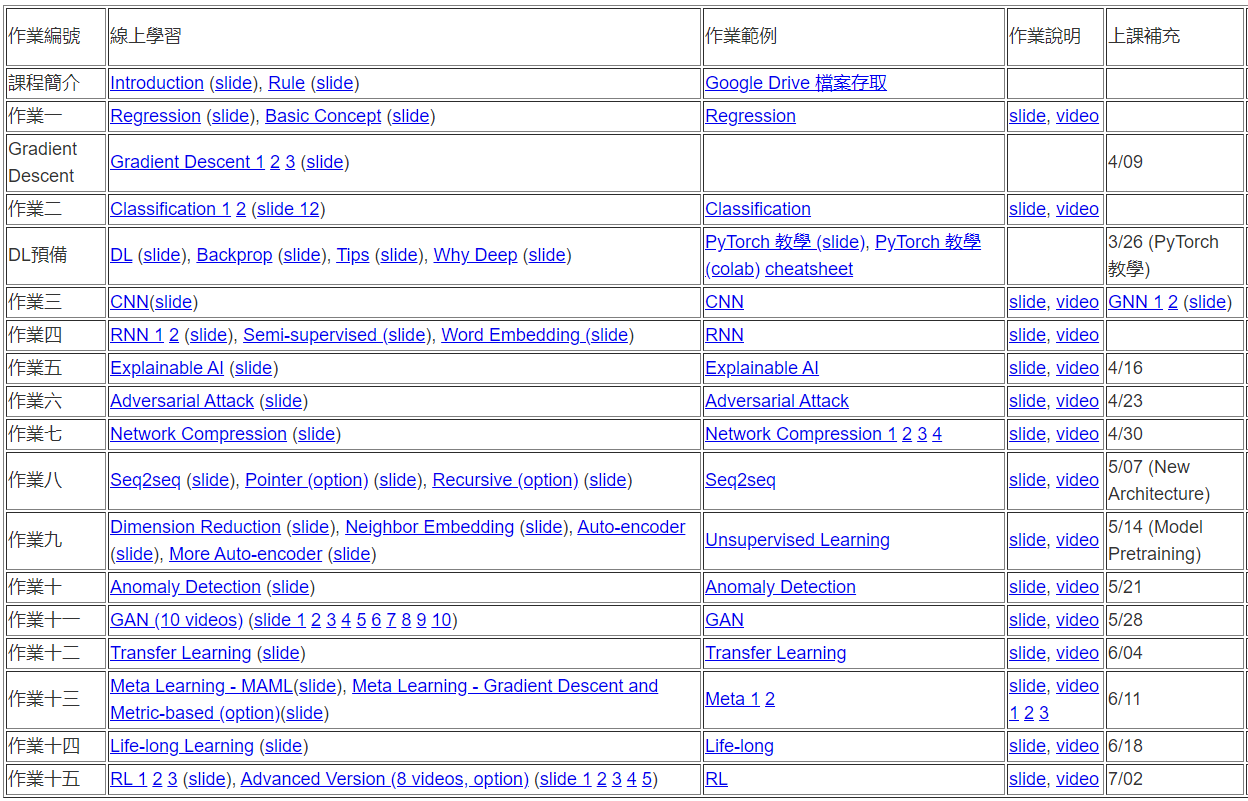在李宏毅的课程首页上的表格，作业、PPT，目前已经全部发布了，还会有助教说明作业的video，如果你打不开网页，请见上方资料3的作业搬运。

作业
博主用的是 win10系统，作业的完成基于 jupyter notebook，博主建议利用 Anaconda 创建的虚拟环境来管理 python 版本和相关库，以免覆盖已有的 python 版本。
相关资料：

Anaconda详细安装及使用教程（带图文）
Anaconda常用命令
Jupyter Notebook介绍、安装及使用教程
链接挂掉后的备用资料：Jupyter Notebook安装和使用详情
如何在jupyter notebook下使用anaconda虚拟环境
pytorch官方教程中文版
pytorch官方教程英文版（打开较慢）

序号
主题
完成情况
完成时间

1
Linear Regression
✔️ 查看详情
2020/04/10

2
Classification
✔️ 查看详情
2020/04/14

3
CNN
✔️ 查看详情
2020/04/26

4
RNN
✔️ 查看详情
2020/07/30

5
Explainable AI
❌

6
❌

7
Network Compression
❌

8
Seq2Seq
❌

9
Unsupervised Learning
❌

10
Anomaly Detection
❌

11
GAN
❌

12
Transfer Learning
❌

13
Meta Learning
❌

14
Life-long Learning
❌

15
Reinforce Learning
❌

笔记
根据b站视频的顺序，简单记录一下每个视频讲了些什么，便于搜索或者跳过。另外，博主会在引用部分中强调一些平时会忽视的点。基本都是2019版及之前的视频，如果有新的，就会标注出来；如果是旧的，就不额外赘述了。
如果你想看视频的文字版，建议去资源4的github学习笔记。

⭐ 李宏毅2020机器学习课程笔记（一）：视频P1-P16的课程梗概
⭐ 李宏毅2020机器学习课程笔记（二）：视频P17-的课程梗概

最后，如果觉得不错，可以小小地赞赏一下（纯自愿）展开全文• 李宏毅 机器学习 PPT 李宏毅 机器学习 PPT机器学习
• 这是台湾李宏毅机器学习视频，是2017年秋季的。包含完整视频PDF格式的PPT。想赚点积分能够去下载一些资源，所以各位请见谅。内是网盘下载地址。
• 台大李宏毅老师机器学习课程课件，全部ppt，官网下载整理机器学习 课件
• 近日，李宏毅上线了 2019 最新版的机器学习课程，授课语言为中文，且全部开放了课程视频。机器学习
• 文件包括李宏毅机器学习和深度学习的入门介绍，包括线性回归、逻辑回归、神经网络等基础知识，也包括从机器学习到深度学习的一个工程，并结合图像识别，详细讲解了机器学习在攻击与防护领域中的应用。 附——作者...
• 李宏毅最新 2020 机器学习课程 http://speech.ee.ntu.edu.tw/~tlkagk/courses_ML20.html 按照作业方式编排； 中文绝佳的 ML/DL 入门课程 视频 + ppt + 作业
• 主要由监督学习、半监督学习、迁移学习、无监督学习、监督学习中的结构化学习以及强化学习构成。最关键的是本文档带书签。机器学习 深度学习
• 李宏毅2020机器学习作业——Linear Regression 一、问题描述 ​ 1. 数据描述 ​ 本次作业采用的数据是使用台湾某一座城市的观测记录，分别为train.csv,test.csv，其中train.csv 共有4320行, 27列，包含了该地点18种...python 机器学习
• 李宏毅2020机器学习课程作业1 平台：windows 编程软件：VScode // A code block # -*- coding: utf-8 -*- import pandas as pd import numpy as np import matplotlib.pyplot as plt import math import csv def ...python 机器学习 numpy
• 开始之前声明：本文参考了李宏毅机器学习作业说明. 欢迎使用Markdown编辑器 你好！ 这是你第一次使用 Markdown编辑器 所展示的欢迎页。如果你想学习如何使用Markdown编辑器, 可以仔细阅读这篇文章，了解一下Markdown...机器学习 深度学习
• 李宏毅机器学习·笔记自己整理成了PDF，现在分享给大家。
• 文章目录机器学习定义机器学习三板斧监督学习Structuerd Learning无监督学习半监督学习Reinforcement LearningTransfer Learning学习导图机器学习开发流程总结 机器学习定义 机器学习三板斧 1.定义一个函数集合 2....人工智能 机器学习
• 李宏毅（Hung-yi Lee）目前任台湾大学电机工程学系和电机资讯学院的助理教授，他曾于 2012 年获得台湾大学博士学位，并于 2013 年赴麻省理工学院（MIT）计算机...他的研究方向主要是机器学习（深度学习）和语音识别。
• 回归Regression一、模型函数二、Goodness of Function损失函数Loss function L：三、挑选出最好的函数梯度下降Gradient Descent 宝可梦CP值预测 设宝可梦的名字为xs、CP值为xcp、HP值为xhp、重量为xw、高度为xh。...
...